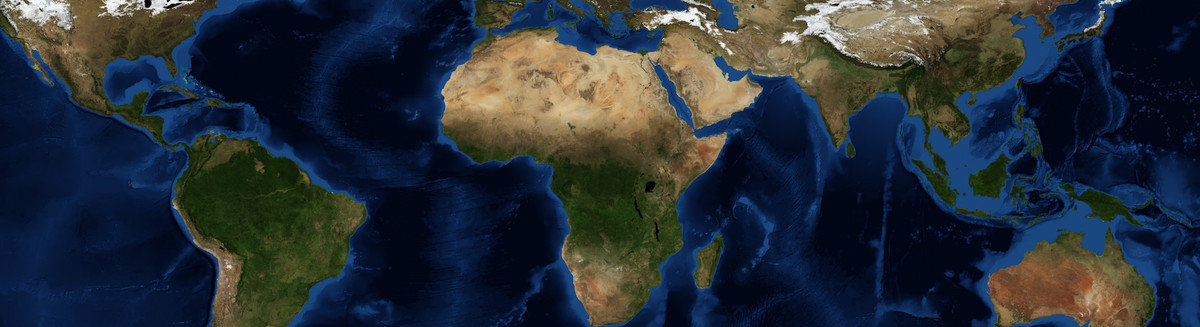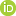This is a Preprint and has not been peer reviewed. The published version of this Preprint is available: https://doi.org/10.1093/gji/ggz277. This is version 2 of this Preprint.

##### Downloads

Download Preprint

##### Authors

Santiago Rubén Soler, Agustina Pesce, Mario E. Gimenez, Leonardo Uieda##### Abstract

We present a new methodology to compute the gravitational fields generated by tesseroids (spherical prisms) whose density varies with depth according to an arbitrary continuous function. It approximates the gravitational fields through the Gauss-Legendre Quadrature along with two discretization algorithms that automatically control its accuracy by adaptively dividing the tesseroid into smaller ones. The first one is a preexisting two dimensional adaptive discretization algorithm that reduces the errors due to the distance between the tesseroid and the computation point. The second is a new density-based discretization algorithm that decreases the errors introduced by the variation of the density function with depth. The amount of divisions made by each algorithm is indirectly controlled by two parameters: the distance-size ratio and the delta ratio. We have obtained analytical solutions for a spherical shell with radially variable density and compared them to the results of the numerical model for linear, exponential, and sinusoidal density functions. The heavily oscillating density functions are intended only to test the algorithm to its limits and not to emulate a real world case. These comparisons allowed us to obtain optimal values for the distance-size and delta ratios that yield an accuracy of 0.1% of the analytical solutions. The resulting optimal values of distance-size ratio for the gravitational potential and its gradient are 1 and 2.5, respectively. The density-based discretization algorithm produces no discretizations in the linear density case, but a delta ratio of 0.1 is needed for the exponential and most sinusoidal density functions. These values can be extrapolated to cover most common use cases, which are simpler than oscillating density profiles. However, the distance-size and delta ratios can be configured by the user to increase the accuracy of the results at the expense of computational speed. Lastly, we apply this new methodology to model the Neuquén Basin, a foreland basin in Argentina with a maximum depth of over 5000 m, using an exponential density function.

##### DOI

https://doi.org/10.31223/osf.io/3548g

##### Subjects

Earth Sciences, Geophysics and Seismology, Physical Sciences and Mathematics

##### Keywords

python, open source, Gravitational fields, Sedimentary Basin, Tesseroids, Variable Density

##### Dates

Published: 2019-06-12 08:48

Last Updated: 2019-06-28 09:35

##### License

CC BY Attribution 4.0 International

Add a Comment

You must log in to post a comment.

Comments

There are no comments or no comments have been made public for this article.